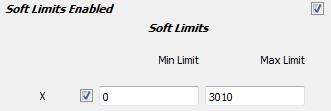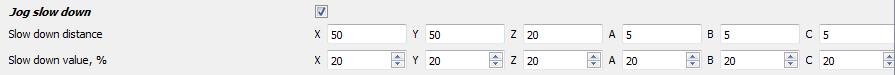mycnc:limits

# Limits

## Soft Limits Enabled

• “Soft Limits Enabled” - this function allows you to set a software limit on the movement of the machine, as well as install and configure hard limit switches for each of the axes.
• To activate the function “Soft Limits Enabled” , it is necessary to check the box next to its name:• To set the limits for each of the axes, you must check the selected axis. The photo shows an example of the choice of this function for the “X”. With the parameters MihLimit = 0 and MaxLimit = 3010, the machine will move along the X axis only within the specified coordinates, i.e. from 0 to 3010.• If there are mechanical limits on the machine, the type of the limit switch and the input number for this switch on the controller is set as shown in the example below.For example, the minus limit sensor is applied to the controller input 5, and the positive limit sensor is applied to the 8 input of the controller. Both shoots are normally closed.
• The sensor type is selected as shown below.• The setting of several axes (X, Y, Z) is given below## Jog slow down

• Function “Jog slow down” - when this function is activated, the machine during the movement will reduce the speed at the entrance to the above specified software limits. The distance at which the speed decreases and the percentage reduction in speed is set in the corresponding table.
• To activate the function “Jog slow down” , it is necessary to check the box next to its name:• The example below shows the setting of the function for several axes (X, Y, Z, A, B)• Taking into account the settings of software limits and settings of the function, the machine will move with a speed reduced to 20% in the coordinates from 0 to 50 (0 + 50) and from 2960 to 3010 (2960 + 50 = 3010). Similarly, when moving along the coordinate Y in coordinates from 0 to 50 (0 + 50) and from 1460 to 1510 (1460 + 50 = 1510) it will move with a speed reduced to 20%.In the same way, when moving along the coordinate Z, in the coordinates from -300 to -280 (-300 + 20 = -280) and from -20 to 0 (0-20 = -20) it will move with a speed reduced to 20%.